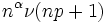# History of Sylow's theorem

Sylow's theorem, originally proved by Ludwig Sylow in 1872, marked an important milestone in the udnerstanding of the general structure of finite groups. This article explores the history behind this theorem and the progress made since then.

## Prior results

### Lagrange's theorem

Further information: Lagrange's theorem, History of Lagrange's theorem

Observations made by Lagrange in the eighteenth century during his investigation of symmetries between roots of the quintic, led to Lagrange's theorem: the order of a subgroup divides the order of the group. This result was well-known for groups of permutations.

### Cauchy's theorem

Cauchy managed to prove a partial converse to Lagrange's theorem. He proved that if$p$ is a prime dividing the order of a finite group$G$, then$G$ has a subgroup of order exactly$p$. The remarkable thing about Cauchy's theorem was that it held for any finite group, and was stated in a language independent of the specific realization of the group as a group of permutations.

To prove this theorem, Cauchy proved that the symmetric group on$n$ letters contains a subgroup of order$p^m$ where$p^m$ is the largest power of$p$ dividing$n!$. This established the existence part of Sylow's theorem for symmetric groups.

## The work of Sylow

Ludwig Sylow, in a paper in 1872, extended Cauchy's result. He proved that any finite group has subgroups of order$p^m$ where$p$ is a prime dividing the order of the group and$p^m$ is the largest power of it dividing the order of the group. Sylow also proved that any two such subgroups, that later came to be called$p$-Sylow subgroups, are conjugate subgroups.

### The introduction of Sylow's paper

Sylow began his paper thus (translated from French):

We know that if the order of a group of substitutions is divisible by the prime number$n$, the group always contains a substitution of order$n$. This important theorem is contained in another, more general theorem, which is: "If the order of a group is divisible by$n^\alpha$,$n$ being prime, the group contains a subgroup of order$n^\alpha$." The demonstration of this theorem provides other general properties of groups of substitutions.

Sylow's first line refers to Cauchy's theorem (at the time of the writing of this paper, bibliography/referencing was not universal), and his second line states the existence part of Sylow's theorem (in fact, it states more: it states that subgroups of all possible prime powers dividing the order exist, but this follows easily from Sylow's theorem and the structure of groups of prime power order).

### His first theorem: Existence and congruence condition (partial)

Theorem I of Sylow's paper reads (translated from French):

If$n^\alpha$ denotes the largest power of the prime number$n$ that divides the order of the group$G$, that group contains another$g$ of order$n^\alpha$; and moreover, if$n^\alpha\nu$ is the order of the big group contained in$G$ comprising those substitutions that permute with$g$, then the order of$G$ is of the form$n^\alpha\nu(np+1)$.

• Sylow's choice of notation is somewhat at odds with current conventions. Sylow uses$n$ for primes, and uses both small and capital letters for sets (thus, calling his subgroup$g$).
• At the time Sylow wrote his paper, terminology like normalizer was not standard; hence, Sylow gave an explicit description for what we'd today simply call$N_G(g)$, the normalizer of$g$ in$G$.
• During Sylow's time, the concept of index of a subgroup was not in wide vogue; hence his statements are all about orders of groups rather than the index of a subgroup in a group.

### His second theorem: Congruence condition and conjugacy

Theorem II of Sylow's paper reads (translated from French):

With all the conditions of the preceding theorem, the group$G$ contains precisely$np + 1$ distinct groups of order$n^\alpha$, one does obtain each by transforming any other by the substitutions in$G$, each group being given by$n^\alpha\nu$ distinct transformations.

• The final clause in his sentence encapsulates the fact that starting with one Sylow subgroup, the ways of conjugating that to another Sylow subgroup correspond to one coset of its normalizer. Each such coset has size$n^\alpha \nu$ -- the size of the normalizer. The language of cosets was not familiar to Sylow; hence he needed to use this wording.